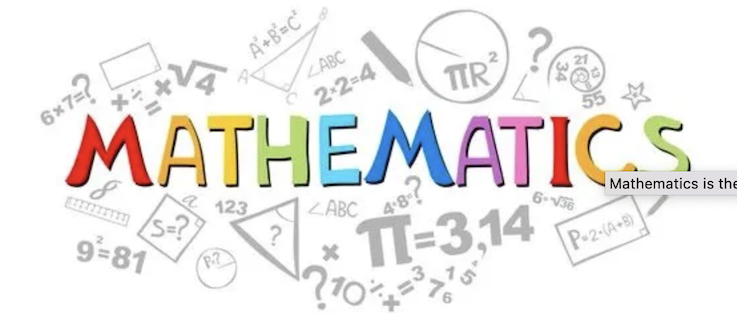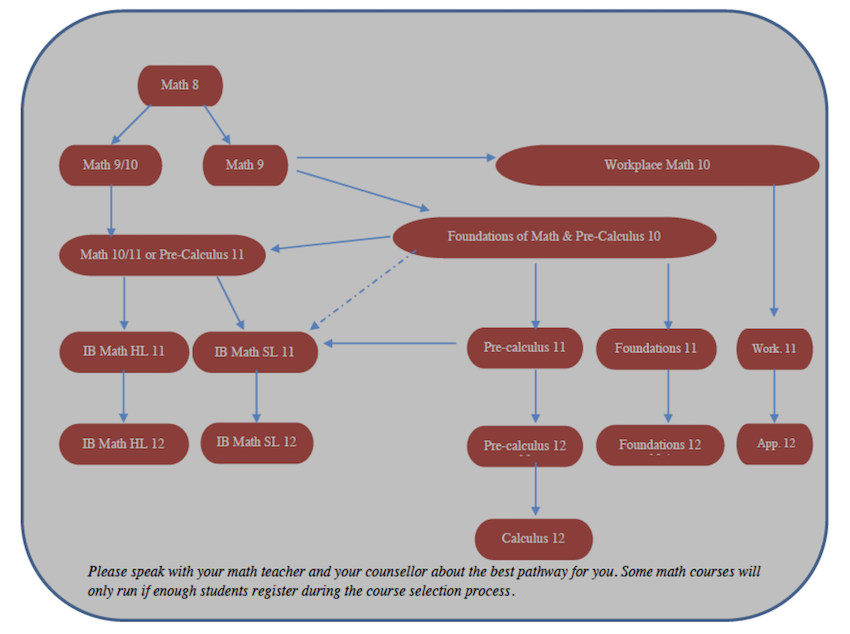# MathematicsMATHEMATICS at RICHMOND SECONDARY SCHOOL

All students will enrol in the same foundational math classes in Grades 8 and 9. Beginning in Grade 10, the math curriculum offers three different pathways. The Workplace Mathematics Pathway is designed to provide students with the mathematical understandings and critical-thinking skills identified for entry in the majority of trades and for direct entry into the work force. The final course on this path is Apprenticeship Math 12. The Foundations of Mathematics Pathway seeks to provide students with the mathematical understandings and critical-thinking skills identified for post-secondary studies in programs that do not require the study of theoretical calculus. The Pre-Calculus Pathway provides students with the mathematical understandings and critical-thinking skills identified for entry into post-secondary programs that require the study of theoretical calculus and sciences. Please check specific post-seconday programs for requirements.

Students entering grade 10 have two options. They may select Workplace Math 10 or Foundations of Mathematics and Pre-Calculus Math 10. Those selecting this second option will then be required to opt for either Foundations of Mathematics 11 or Pre-calculus Mathematics 11 beginning in their grade 11 year.

For highly motivated students wanting to pursue mathematics that offers a greater challenge, consider accelerated courses like Math 9/10 and Math 10/11 for preparation to enter IB Mathematics in our International Baccalaureate Diploma Program.  IB Math requires students to achieve a high standing in Foundations and Pre-Calculus Math 10 and it is strongly recommended that students complete Pre-Calculus 11 prior to enrolling in IB Math courses.

Remember that a student’s math teacher and counsellor have spent a lot of time working with that student and are able to give realistic advice based on a student’s ability and interest in math.MATHEMATICS 08
Math 8 is now using a new B.C. curriculum.  Students will be learning some big ideas of mathematics which include numbers, numeracy, patterns, computational fluency, geometry, data analysis and problem solving. Details can be found at: https://curriculum.gov.bc.ca/curriculum/mathematics/8

MATHEMATICS 09
Math 9 is now using a new B.C. curriculum.  Students will be learning some big ideas of mathematics which include numbers, numeracy, patterns, computational fluency, geometry, data analysis and problem solving.  Details can be found at: https://curriculum.gov.bc.ca/curriculum/mathematics/9

MATHEMATICS 9/10
Admission is restricted to students who have been identified through the Math 9/10 entrance exam and by Grade 8 teachers as appropriate for a more challenging environment. Enrolment is limited and will be determined by the Math Department. This course is designed for motivated students with highly developed math skills. It is a fast paced course that will cover Math 9 and Math 10 topics.

WORKPLACE MATHEMATICS 10
Recommended: Mathematics 9 and consultation with students and parent.
The purpose of this course is to introduce students to the mathematical understandings and critical-thinking skills necessary for entry into the majority of trades and for direct entry into the workforce. Topics include unit pricing, currency exchange, income, measurement, geometry and trigonometry. Please note, the Workplace math pathway is not accepted by most university programs.

FOUNDATIONS OF MATHEMATICS AND PRE-CALCULUS 10
This course is the common starting point for the Foundations of Mathematics and Pre-calculus pathways. Its purpose is to begin to provide a conceptual knowledge base and skill set necessary for students whose future interests include post-secondary studies. Upon completion of this course, students can opt for the Pre-calculus pathway if their post-secondary plans require the study of theoretical calculus or the Foundations pathway for programs that do not. Topics include trigonometry, finance, algebra, relations and functions, linear equations and systems.

MATHEMATICS 10/11 (NOT OFFERED IN 2022-2023)
Students must have taken Mathematics 9/10 and have permission from the Math Department. Students currently enrolled in Mathematics 9/10 will not be guaranteed entrance into this course. This course is a continuation of Mathematics 9/10. This course covers the same curriculum as Pre-calculus 11, but to a greater degree of depth and enrichment. This course is intended for students wishing to enter IB Math.

WORKPLACE MATHEMATICS 11
Recommended: Workplace Math 10 or teacher recommendation
This course is a continuation of Workplace Math 10. The purpose is to introduce students to the mathematical understandings and critical-thinking skill necessary for entry into the majority of trades and for direct entry into the workforce. Topics include slope, graphs, measurement, trigonometry, scale diagrams, finance and budgeting.

FOUNDATIONS OF MATH 11
This pathway is designed to provide students with the mathematical understandings and critical-thinking skills identified for post-secondary studies in programs that do not require the study of theoretical calculus. Topics include measurement, geometry, logical reasoning, statistics, relations and functions and a mathematics research project. It is recommended that students check and confirm with their post-secondary plans for acceptance of the Foundations math pathway.

PRE-CALCULUS 11
Recommended: Foundations of Mathematics and Pre-calculus 10 with at least a high C+ average
This pathway is designed to provide students with the mathematical understandings and critical thinking skills identified for entry into post-secondary program that require the study of theoretical calculus. Topics include sequences and series, rational expressions, trigonometry, factoring, quadratric/radical functions and equations, systems of equations and inequalities.

PRE-CALCULUS 12
Recommended: Pre-calculus 11 (with at least a high C+ average)
This course is a continuation of Pre-calculus 11. It is designed for students who are entering post-secondary programs that require the study of theoretical calculus. Please check specific program requirements. Topics include trigonometry, logarithms, conics, sequences and series and polynomials.

FOUNDATIONS OF MATH 12
Recommended: Foundations 11 with a C+ average
This course is a continuation of Foundations 11. It is designed for students who are entering post-secondary programs THAT DO NOT require the study of theoretical calculus. Please check specific program requirements. Topics include financial math, set theory and logic, counting methods, probability, polynomial/exponential/logarithmic/ sinusoidal functions and a mathematics research project.

APPRENTICESHIP MATH 12
Recommended: Workplace Math 11
This course is a continuation of Workplace Math 11. It is designed for students going directly into the workforce or into trades programs. Topics include measurement, geometry, number, algebra, statistics and probability.

CALCULUS 12
Recommended: Pre-calculus 12 (with a high B average) OR IB Mathematics (SL) 11 (a high B average)
This challenging course is intended for post-secondary institution bound students who will be required to take a calculus course. The curriculum consists of limits, differentiation, applications of the derivative, curve sketching, trigonometric, logarithmic and exponential differentiation, antiderivatives, differential equations, and areas and integrals. A graphing calculator is required for this course.

Mathematics in the International Baccalaureate Diploma Programme is designed for students with a genuine interest in math and a willingness to extend their thinking, computational and problem solving abilities. IB math is challenging and it is expected that you already have solid mathematical knowledge and skills and have successfully completed Foundations and Pre-Calculus Math 10 and it is strongly recommended that you have already completed Pre-Calculus Math 11.  Our teachers are dedicated and will both challenge and support you, but you must be a hard working and resilient learner to be successful in IB math.

IB MATHEMATICS ANALYSIS AND APPROACHES SL 11
Standard Level IB Mathematics is a challenging course. It is strongly recommended that students have a high standing in Foundations and Pre-Calculus Math 10 and it is strongly advised that students have also completed Pre-calculus Math 11. Students will have an interview at RSS and achieve a satisfactory standing in an entrance exam. This single-blocked course is designed to provide students of high mathematical ability and good work habits with challenging materials. Topics include algebra, functions and equations, geometry and trigonometry and the beginning of a mathematical exploration.  Students receive credit for IB Math SL 11.  A graphing calculator is required for this course.

IB MATHEMATICS ANALYSIS AND APPROACHES HL 11
This very challenging higher level mathematics course is double blocked and will be available to only 30 students entering Grade 11.
Students selecting HL Mathematics must have completed Math 10/11 OR Pre-calculus 11 (with a very high standing), attend an interview, achieve a satisfactory standing in an entrance exam, and permission by the Math Department. Please note that this is the first year of a very challenging two-year course and the class will meet every day all year. It is designed for students with a genuine passion for math, who possess high mathematical thinking abilities and are resilient, dedicated students with excellent work habits and plan to complete the HL exam in Grade 12. Topics include algebra, functions and equations, circular functions and trigonometry, matrices, vectors, statistics and probability, calculus and optional topics, mathematical investigations and models.  Students will receive their IB Math HL  11 credit.  A graphing calculator is required for this course.

IB MATHEMATICS ANALYSIS AND APPROACES SL 12
Prerequisite: IB Mathematics SL 11

This course is a continuation of IB Mathematics SL 11. The core curriculum consists of probability, statistics, calculus and vectors and completion of a mathematical exploration.  The IB SL Math exam will be written in May of the Grade 12 year.

IB MATHEMATICS ANALYSIS AND APPROACES HL 12
Prerequisite: IB Mathematics HL 11
This course is a continuation of IB Mathematics HL 11. The core curriculum consists of mathematical induction, complex numbers, vector algebra, 3D geometry, transformations, probability and statistics and further calculus. The IB HL Math exam will be written in May of the Grade 12 year.